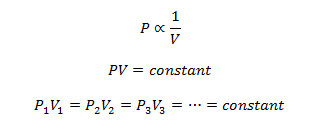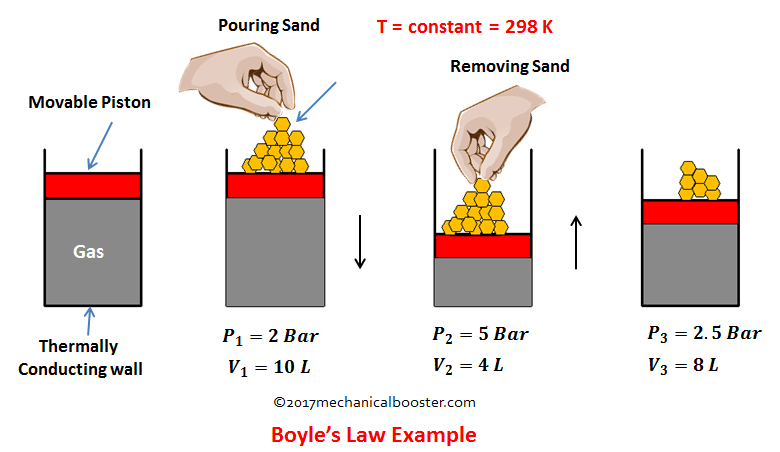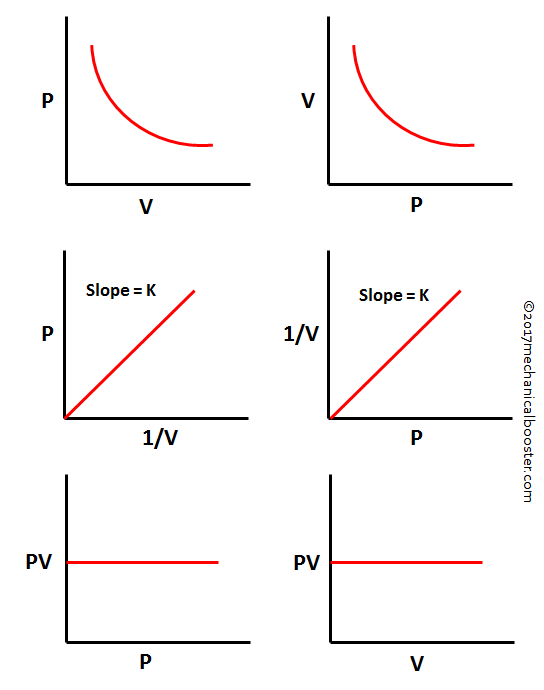# Boyle’s Law – Statement, Formula Example and Graph

## What is Boyle’s Law?

This law was established by Robert Boyle in 1662.

At constant temperature, the absolute pressure of a given mass of a perfect gas is inversely proportional to its volume.

Have you understand something from this? If not than let’s we understand what this statement implies. It means that if we have a perfect gas of a given mass at constant temperature (i.e. the temperature which remains unchanged during the change of state of the gas), and if the pressure of the gas increases than the volume of the gas decreases and if the pressure decreases than the volume of the gas increases. So the conclusion is that the pressure varies inversely to its volume at constant temperature for a perfect gas.

MathematicallyHere the suffixes 1, 2, 3 represents different sets of conditions.

## Example of Boyle’s LawTo understand this law more clearly let’s take an example. Suppose we have a system of cylinder piston arrangement in which a perfect gas of given mass is present and the piston is free to slide in the cylinder. Let the system is place at room temperature and initially P1, V1 be the pressure and volume of the gas. Now start putting some sand gradually on the upper part of piston. What you will see from this, you will observe that the force on the piston increases and so the pressure and this pushes the piston downward. The piston starts moving downward results in decrease of the volume. Here the increase in pressure results in decrease in volume. Now start removing the sand gradually, since we are removing the sand, so we are decreasing the pressure on the gas. This time we see that the piston moves upward and the volume of the gas increases. From this the conclusion comes out is that as the pressure on the gas increases its volume decreases and when pressure on the gas decreases than the volume increases.

In the above example if we calculate the value of PV in each case, we will get the same answer i.e. 20.

Note: Since the system is at room temperature so the temperature of the system remains constant ( T = 298 K) in every state change. A confusion arises with that, how this temperature remains constant when we compresses the gas because on compression the temperature should be increases. Yes your confusion is right. As the piston gradually compresses the gas, the temperature of the gas increases but the extra increases in temperature is rejected to the surrounding as heat through the conducting walls the cylinder. And the temperature of the gas remains unchanged.

The above example proves the statement of the Boyle’s law.

## Graph

The various graph of the Boyle’s law is given below: